# Logical Reasoning and Analytical Ability (Test 5)

## Upsc Civil Services Entrance Exams : General Studies Test

| Home | | Upsc Civil Services Entrance Exams | | General Studies Test | | Logical Reasoning and Analytical Ability |Logical Reasoning and Analytical Ability
| Logical Reasoning | | Analytical Reasoning |
Q.1
Find the number of triangles in the given figure.A. 18
B. 20
C. 24
D. 27
Explaination / Solution:

The figure may be labelled as shown.The simplest triangles are IJO, BCJ, CDK, KQL, MLQ, GFM, GHN and NIO i.e. 8 in number.

The triangles composed of two components each are ABO, AHO, NIJ, IGP, ICP, DEQ, FEQ, KLM, LCP and LGP i.e.10 in number.

The triangles composed of four components each are HAB, DEF, LGI, GIC, ICL and GLC i.e. 6 in number.

Total number of triangles in the figure = 8 + 10 + 6 = 24.

Workspace
Report
Q.2
Find the number of triangles in the given figure.A. 16
B. 13
C. 9
D. 7
Explaination / Solution:

The figure may be labelled as shown.The simplest triangles are AGE, EGC, GFC, BGF, DGB and ADG i.e. 6 in number.

The triangles composed of two components each are AGC, BGC and ABG i.e. 3 in number.

The triangles composed of three components each are AFC, BEC, BDC, ABF, ABE and DAC i.e. 6 in number.

There is only one triangle i.e. ABC composed of six components.

Thus, there are 6 + 3 + 6 + 1 = 16 triangles in the given figure.

Workspace
Report
Q.3
Find the number of triangles in the given figure.A. 10
B. 12
C. 14
D. 16
Explaination / Solution:

The figure may be labelled as shown.The simplest triangles are ABJ, ACJ, BDH, DHF, CIE and GIE i.e. 6 in number.

The triangles composed of two components each are ABC, BDF, CEG, BHJ, JHK, JKI and CJI i.e. 7 in number.

There is only one triangle JHI which is composed of four components.

Thus, there are 6 + 7 + 1 = 14 triangles in the given figure.

Workspace
Report
Q.4

Find the number of triangles in the given figure.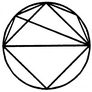A. 8
B. 10
C. 11
D. 12
Explaination / Solution:

The figure may be labelled as shown.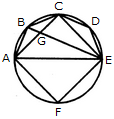The simplest triangles are ABG, BCG, CGE, CDE, AGE and AEF i.e. 6 in number.

The triangles composed of two components each are ABE, ABC, BCE and ACE i.e. 4 in number.

There are 6 + 4 = 10 triangles in the figure.

Workspace
Report
Q.5

What is the number of straight lines and the number of triangles in the given figure.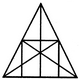A. 10 straight lines and 34 triangles
B. 9 straight lines and 34 triangles
C. 9 straight lines and 36 triangles
D. 10 straight lines and 36 triangles
Explaination / Solution:

The figure may be labelled as shown.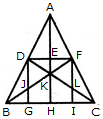The Horizontal lines are DF and BC i.e. 2 in number.

The Vertical lines are DG, AH and FI i.e. 3 in number.

The Slanting lines are AB, AC, BF and DC i.e. 4 in number.

Thus, there are 2 + 3 + 4 = 9 straight lines in the figure.

Now, we shall count the number of triangles in the figure.

The simplest triangles are ADE, AEF, DEK, EFK, DJK, FLK, DJB, FLC, BJG and LIC i.e. 10 in number.

The triangles composed of two components each are ADF, AFK, DFK, ADK, DKB, FCK, BKH, KHC, DGB and FIC i.e. 10 in number.

The triangles composed of three components each are DFJ and DFL i.e. 2 in number.

The triangles composed of four components each are ABK, ACK, BFI, CDG, DFB, DFC and BKC i.e. 7 in number.

The triangles composed of six components each are ABH, ACH, ABF, ACD, BFC and CDB i.e. 6 in number.

There is only one triangle i.e. ABC composed of twelve components.

There are 10 + 10 + 2 + 7 + 6+ 1 = 36 triangles in the figure.

Workspace
Report
Q.6

Find the number of triangles in the given figure.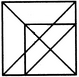A. 16
B. 18
C. 19
D. 21
Explaination / Solution:

The figure may be labelled as shown.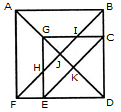The simplest triangles are EFH, BIC, GHJ, GIJ, EKD and CKD i.e. 6 in number.

The triangles composed of two components each are ABJ, AFJ, GCK, GEK, CED arid GHI i.e. 6 in number.

The triangles composed of three components each are GCD, GED, DJB and DJF i.e. 4 in number.

The triangles composed of four components each are ABF and GCE i.e. 2 in number.

The triangles composed of five components each are ABD and AFD i.e. 2 in number.

There is only one triangle i.e. FBD composed of six components.

Total number of triangles in the figure = 6 + 6 + 4 + 2 + 2 + 1 = 21.

Workspace
Report
Q.7

What is the minimum number of colours required to fill the spaces in the given diagram without any two adjacent spaces having the same colour?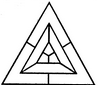A. 6
B. 5
C. 4
D. 3
Explaination / Solution:

The figure may be labelled as shown.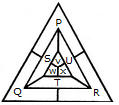The spaces P, Q and R have to be shaded by three different colours definitely (since each of these three spaces lies adjacent to the other two).

Now, in order that no two adjacent spaces be shaded by the same colour, the spaces T, U and S must be shaded with the colours of the spaces P, Q and R respectively.

Also the spaces X, V and W must be shaded with the colours of the spaces S, T and U respectively i.e. with the colours of the spaces R, P and Q respectively. Thus, minimum three colours are required.

Workspace
Report
Q.8

Count the number of parallelogram in the given figure.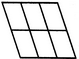A. 20
B. 18
C. 16
D. 12
Explaination / Solution:

The figure may be labelled as shown.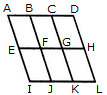The simplest ||gms are ABFE, BCGF, CDHG, EFJI, FGKJ and GHLK. These are 6 in number.

The parallelograms composed of two components each are ACGE, BDHF, EGKI, FHLJ, ABJI, BCKJ and CDLK. Thus, there are 7 such parallelograms.

The parallelograms composed of three components each are ADHE and EHLI i.e. 2 in number.

The parallelograms composed of four components each are ACKI and BDLJ i.e. 2 in number

There is only one parallelogram composed of six components, namely ADLI.

Thus, there are 6 + 7 + 2 + 2 + 1 = 18 parallelograms in the figure.

Workspace
Report
Q.9

Count the number of squares in the given figure.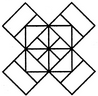A. 22
B. 20
C. 18
D. 14
Explaination / Solution:

The figure may be labelled as shown.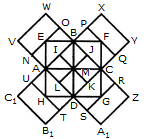The squares composed of two components each are BJMI, CKMJ, DLMK and AIML i.e. 4 in number.

The squares composed of three components each are EBMA, BFCM, MCGD and AMDH i.e. 4 in number.

The squares composed of four components each are VWBA, XYCB, ZA1DC and B1C1AD i.e. 4 in number.

The squares composed of seven components each are NOJL, PQKI, RSLJ and TUIK i.e. 4 in number.

There is only one square i.e. ABCD composed of eight components.

There is only one square i.e. EFGH composed of twelve components.

Total number of squares in the figure = 4 + 4 + 4 + 4 + 1 + 1 = 18.

Workspace
Report
Q.10

Determine the number of rectangles and hexagons in the given figure.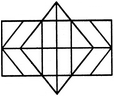A. 30, 5
B. 32, 3
C. 28, 5
D. 30, 3
Explaination / Solution:

The figure may be labelled as shown.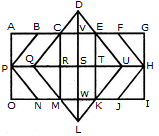Rectangles:

The simplest rectangles are CVSR, VETS, RSWM and STKW i.e. 4 in number.

The rectangles composed of two components each are CETR, VEKW, RTKM and CVWM i.e. 4 in number.

The rectangles composed of three components each are ACRP, PRMO, EGHT and THIK i.e. 4 in number.

The rectangles composed of four components each are CEKM, AVSP,PSWO, VGHS and SHIW i.e. 5 in number.

The rectangles composed of five components each are AETP, PTKO, CGHR and RHIM i.e. 4 in number.

The rectangles composed of six components each are ACMO and EGIK i.e. 2 in number.

The rectangles composed of eight components each are AGHP, PHIO, AVWO and VGIW i.e. 4 in number.

The rectangles composed of ten components each are AEKO and CGIM i.e. 2 in number.

AGIO is the only rectangle having sixteen components.

Total number of rectangles in the given figure

= 4 + 4 + 4 + 5 + 4 + 2 + 4 + 2 + 1 = 30.

Hexagons:

The hexagons in the given figure are CDEKLM, CEUKMQ, CFHJMQ, BEUKNP and BFHJNP.

So, there are 5 hexagons in the given figure.

Workspace
Report